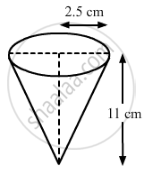Share

# Sushant has a vessel, of the form of an inverted cone, open at the top, of height 11 cm and radius of top as 2.5 cm and is full of water. Metallic spherical balls each of diameter 0.5 - CBSE Class 10 - Mathematics

ConceptSurface Areas and Volumes Examples and Solutions

#### Question

Sushant has a vessel, of the form of an inverted cone, open at the top, of height 11 cm and radius of top as 2.5 cm and is full of water. Metallic spherical balls each of diameter 0.5 cm are put in the vessel due to which 2/th of the water in the vessel flows out. Find how many balls were put in the vessel. Sushant made the arrangement so that the water that flows out irrigates the flower beds. What value has been shown by Sushant?

#### SolutionHeight (h) of the conical vessel = 11 cm
Radius (r1) of the conical vessel = 2.5 cm
Radius (r2) of the metallic spherical balls =0.5/2 =0.25 cm
Let n be the number of spherical balls that were dropped in the vessel.
Volume of the water spilled = Volume of the spherical balls dropped

2/5xx"Volume of cone" = nxx"Volume of one spherical ball"

⇒2/5xx1/3πr_1^2h = nxx4/3πr_2^3

⇒r_1^2h=nxx10r_2^3

⇒(2.5)^2×11=n×10×(0.25)^3

⇒68.75=0.15625n

⇒n=440

Hence, the number of spherical balls that were dropped in the vessel is 440.
Sushant made the arrangement so that the water that flows out, irrigates the flower beds. This shows the judicious usage of water.

Is there an error in this question or solution?

#### APPEARS IN

RD Sharma Solution for 10 Mathematics (2018 to Current)
Chapter 14: Surface Areas and Volumes
Ex. 14.10 | Q: 47 | Page no. 30

#### Video TutorialsVIEW ALL 

Solution Sushant has a vessel, of the form of an inverted cone, open at the top, of height 11 cm and radius of top as 2.5 cm and is full of water. Metallic spherical balls each of diameter 0.5 Concept: Surface Areas and Volumes Examples and Solutions.
S